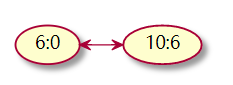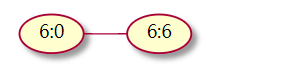# 剑指Offer-03.数组中重复的数字

``````

[2, 3, 1, 0, 2, 5, 3]

``````

``````
2 <= n <= 100000

``````

## 一解

``````
class Solution:

def findRepeatNumber(self, nums: List[int]) -> int:

tmp = []

for num in nums:

if num not in tmp:

tmp.append(num)

# 如果该数在 tmp 中，就确认有重复

else:

return num

``````
• 执行用时：5692ms，在所有 Python3 提交中击败了 7.74%的用户

• 内存消耗：23.5MB，在所有 Python3 提交中击败了 27.25%的用户

• 使用临时数组消耗大量内存

• `if num not in tmp`的 in 操作是 O(n) ，浪费时间

## 二解``````
class Solution:

def findRepeatNumber(self, nums: List[int]) -> int:

n = len(nums)

for i in range(n):

# 只有 i != nums[i] 时，才说明要判断的两个元素在两个位置，即 nums[i] 和 nums[nums[i]] 不是同一个元素

if i != nums[i]:

if nums[i] == nums[nums[i]]:

return nums[i]

tmp = nums[i]

nums[i], nums[tmp] = nums[tmp], nums[i]

``````
• 执行用时：32ms，在所有 Python3提交中击败了99.79%的用户

• 内存消耗：23.4MB，在所有 Python3提交中击败了52.83%的用户

class Solution():
def findRepeatNumber(self, nums):
lenth=len(nums)
while (lenth>0):
number=nums.pop()
nums=nums
if (number in nums)==True:
return number
break
lenth=len(nums)
return None